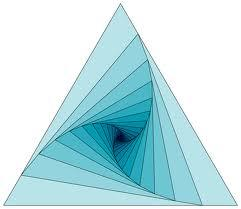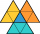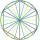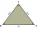# Sides of triangle

Triangle has circumference 42 cm. Side a is 2 times shorter than side b and sice c is 2 cm longer than side a. Determine the sizes of sides of a triangle.

a =  16
b =  8
c =  18

### Step-by-step explanation:

a+b+c=42
a=2b
c = a+2

a+b+c=42
a=2•b
c = a+2

a+b+c = 42
a-2b = 0
a-c = -2

a = 16
b = 8
c = 18

Our linear equations calculator calculates it.Did you find an error or inaccuracy? Feel free to write us. Thank you!Tips to related online calculators
Do you have a linear equation or system of equations and looking for its solution? Or do you have a quadratic equation?

#### You need to know the following knowledge to solve this word math problem:

We encourage you to watch this tutorial video on this math problem:

## Related math problems and questions:

• Three sidesSide b is 2 cm longer than side c, side a is 9 cm shorter than side b. The triangle circumference is 40 cm. Find the length of sides a, b, c . .. .
• Triangle ABCIn a triangle ABC side b measure 10 cm less than the side a and side b is half of the side c. Calculate the length of sides if the circumference of the triangle is 42 cm.
• Triangle - is RT?Triangle has a circumference of 90 cm. Side b is 1 cm longer than c, and side c is 31 cm longer than side a. Calculate the length of sides and determine whether a triangle is a right triangle.
• Two similarTwo similar triangles, one has a circumference of 100 cm, the second has sides successively 8 cm, 14 cm, 18 cm longer than the first. Find the lengths of its sides.
• Arm and baseThe isosceles triangle has a circumference of 46 cm. Calculate its area if the arm is 5 cm longer than the base.
• Isosceles trapezoidPerimeter of the isosceles trapezoid is 48 cm. One side is two times greater than the second side. Determine the dimensions of the trapezoid.
• RT perimeterThe leg of the rectangular triangle is 7 cm shorter than the second leg and 8 cm shorter than the hypotenuse. Calculate the triangle circumference.
• Rectangle - sidesWhat is the perimeter of a rectangle with area 266 cm2 if length of the shorter side is 5 cm shorter than the length of the longer side?
• ParallelogramThe perimeter of the parallelogram is 417 cm. The length of one side is 1.7-times longer than the length of the shorter side. What is the length of sides of a parallelogram?
• The rectangleThe rectangle has a circumference of 32 m. One side is 2 m longer than the other side. Calculate the side lengths of this rectangle.
• Rectangles - sidesOne side of the rectangle is 10 cm longer than a second. Shortens longer side by 6 cm and extends shorter by 14 cm increases the rectangle area by 130 cm2. What are the dimensions of the original rectangle?
• Right triangle eq2Hypotenuse of a right triangle is 9 cm longer than one leg and 8 cm longer than the second leg. Determine the circumference and area of a triangle.
• Polygon 42Which polygon has 42 more diagonals than sides?
• Side lengthsIn the triangle ABC, the height to the side a is 6cm. The height to side b is equal to 9 cm. Side "a" is 4 cm longer than side "b". Calculate the side lengths a, b.
• ParallelogramSide a of parallelogram is twice longer than side b. Its circumference is 78 dm. Calculate the length of the sides of a parallelogram.
• Sides of triangleTriangle circumference with two identical sides is 117cm. The third side measures 44cm. How many cms do you measure one of the same sides?
• Rectangle vs squareOne side of the rectangle is 1 cm shorter than the side of the square, the second side is 3 cm longer than the side of the square. Square and rectangle have the same content. Calculate the length of the sides of a square and a rectangle.